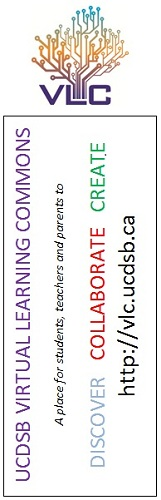# Grade 11-12 Physics: Gravitational, Electric, and Magnetic Fields

## VLC Bookmark

Logins for all VLC databases are found on the back of the VLC Bookmark.## Understanding Basic Concepts

Terms related to gravitational, electric, and magnetic fields include:

• forces
• potential energies
• potential
• exchange particles
• Newton's law of universal gravitation and circular motion
• electric force
• field strength
• uniform and non-uniform electric fields
• gravitational fields
• uniform magnetic field
• near-Earth and distant fields
• parallel plate
• point charges
• Coulomb's law
• Millikan's experiment
• Rutherford's scattering experiment
• the theory of general relativity
• straight-line conductors
• solenoids
• standard model of particle physics